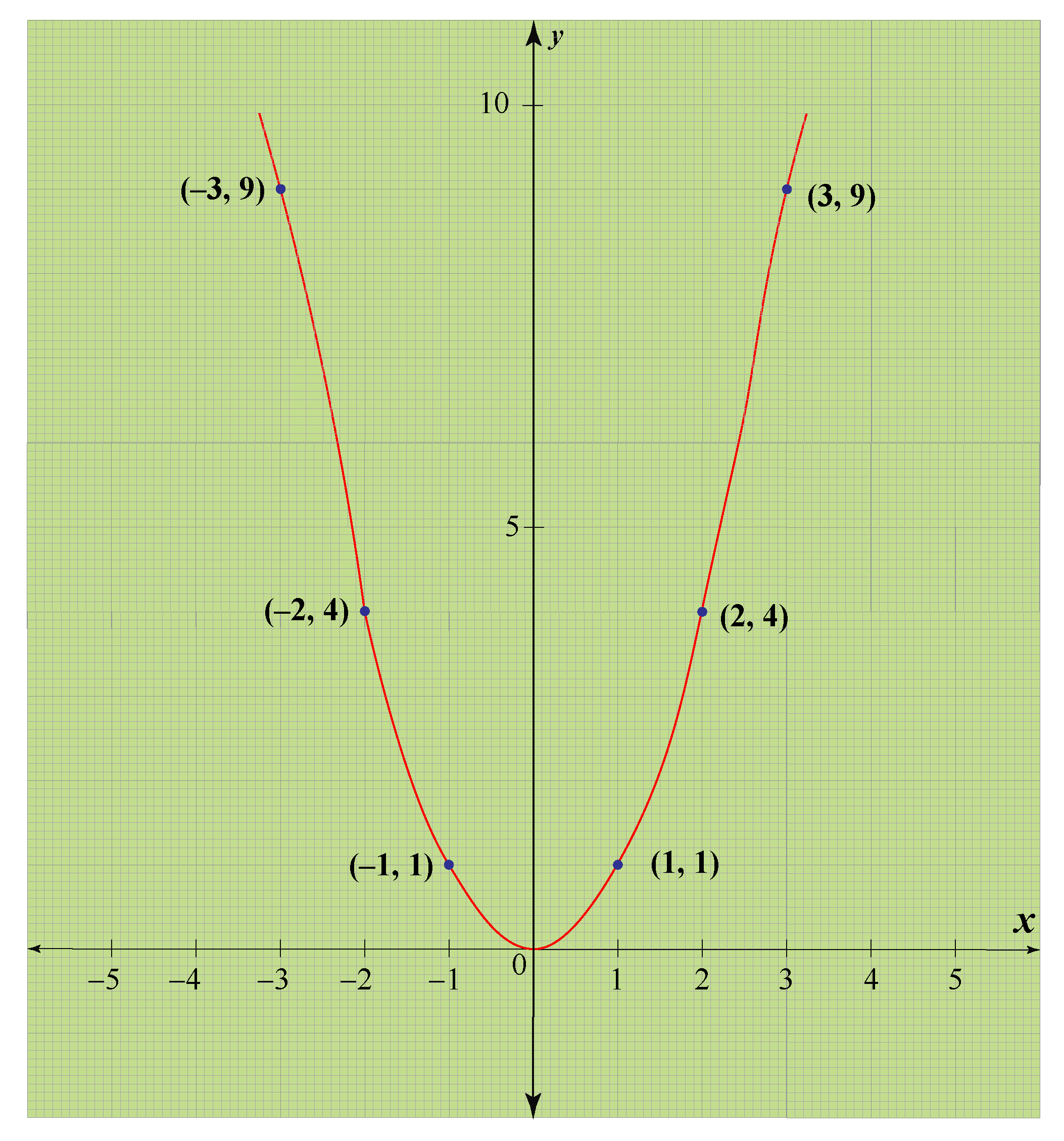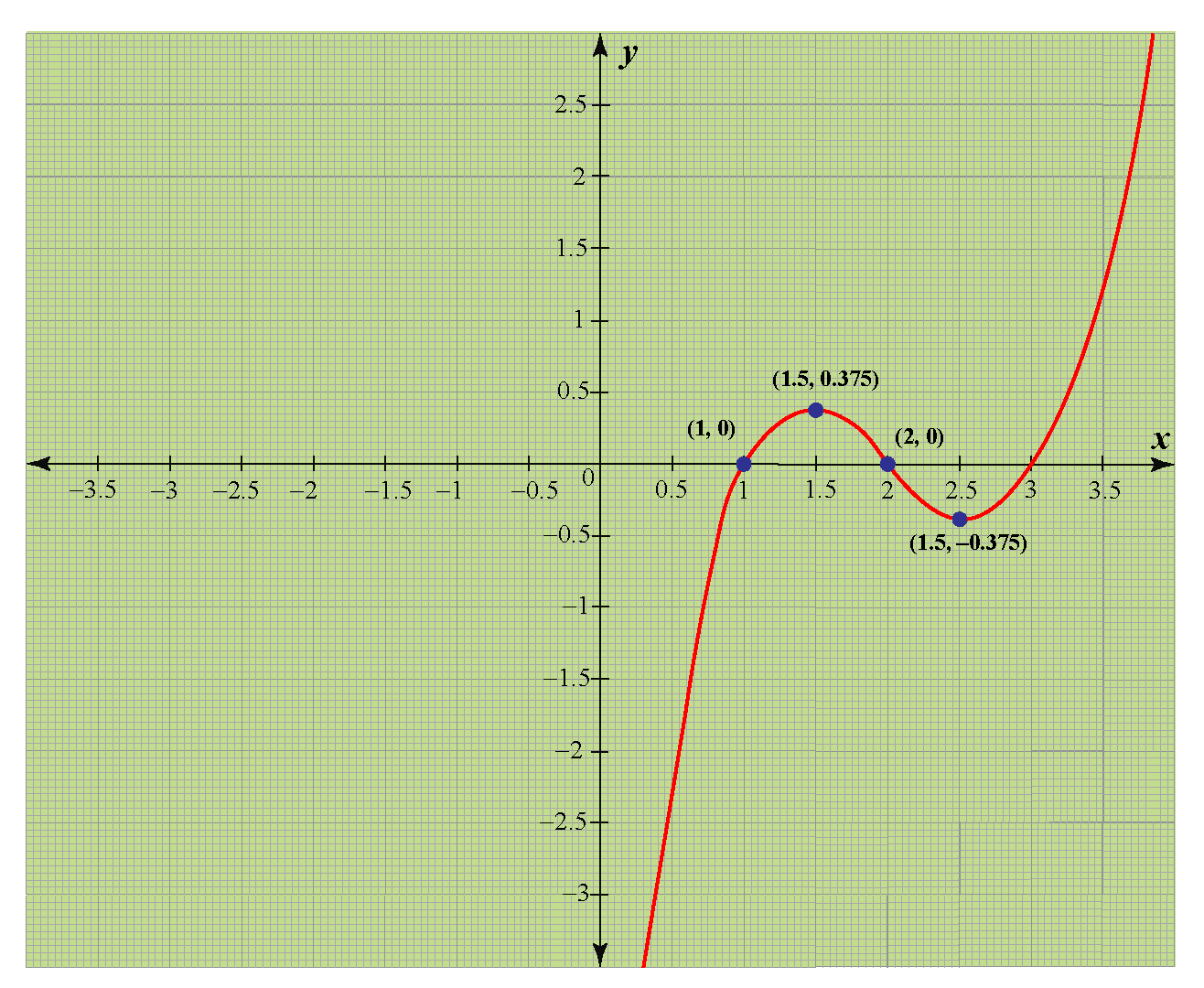# Value of a Polynomial

Value of a Polynomial

In this mini-lesson, we will learn about the value of a polynomial by understanding its meaning, and we will also understand how to find the value of a polynomial expression.

We know that a polynomial contains variables, constant terms, and operators.

A polynomial is a mathematical expression written in the form:

$a_0x^n + a_1x^{n-1} + a_2x^{n-2}...........+ a_nx^0$

The above expression is also called "polynomials in the standard form."

Where $$a_0, a_1, a_2.........a_n$$ are constants and $$n$$ is a natural number.

If we change the value of the variable, the value of the polynomial also changes.

For example, if the value of $$x$$ in the polynomial $$P(x) = x + 1$$ changed from $$x = 1$$ to $$x = 2$$ the value of $$P(x)$$ also changes from $$2$$ to $$3$$

Let's consider the graph of the function $$y = x^2$$We can clearly see that the graph is taking different values for different values of $$x$$.

Now, let's explore the value of a polynomial in more detail.

## Lesson Plan

 1 What do you Mean by Value of a Polynomial? 2 Thinking Out of the Box! 3 Solved Examples 4 Important Notes on Value of a Polynomial 5 Interactive Questions

## What do you Mean by Value of a Polynomial?

The value of a polynomial means the value a polynomial takes if we substitute the variables with any number.

For example, $$P(x)$$ is a polynomial; the value $$P(x)$$ takes at any $$x = a$$ is $$P(a)$$

 Value of $$P(x)$$ at $$x = a$$ is $$P(a)$$

To find the value of $$P(x)$$ at $$x = a$$, we need to replace $$x$$ with $$a$$ in the polynomial.

Let's consider the graph of the function $$y = x^3 - 6x^2 + 11x - 6$$Now, we can clearly observe that the graph of $$y = x^3 - 6x + 11x - 6$$ is taking different values at different values of $$x$$.

At $$x = 1$$, $$y = 0$$
At $$x = 1.5$$, $$y = 0.375$$
At $$x = 2$$, $$y = 0$$
At $$x = 2.5$$, $$y = -0.375$$

Enter the polynomial and explore the graph of the polynomial to check for the value of $$y$$ at any value of $$x$$.Think Tank
• If a polynomial goes to negative infinity when $$x$$ goes to negative infinity, and positive infinity when $$x$$ goes to positive infinity, will this polynomial ever take a zero value at some $$x$$? If it can take, then can we find the number of values of $$x$$ at which the polynomial takes zero value? Think about it.

## How to Find the Value of a Polynomial Expression?

To find the value of a polynomial at a point $$x = a$$, we only need to replace $$x$$ with $$a$$ in the equation of the polynomial.

So, if $$P(x)$$ is a polynomial, the value $$P(x)$$ takes at any $$x = a$$ is $$P(a)$$.

Let's consider an example.

If $$P(x) = x^2 + x + 1$$, then the value of $$P(x)$$ at different values of $$x$$ can be calculated as follows:

At $$x = 0$$, it means that we have to find the value of $$P(0)$$.

So, replace $$x$$ with $$0$$.

$$P(0) = 0^2 + 0 + 1 = 0 + 0 + 1 = 1$$

At $$x = 1$$, it means that we have to find the value of $$P(1)$$.

So, replace $$x$$ with $$1$$.

$$P(1) = 1^2 + 1 + 1 = 1 + 1 + 1 = 3$$

At $$x = 2$$, it means that we have to find the value of $$P(2)$$.

So, replace $$x$$ with $$2$$.

$$P(2) = 2^2 + 2 + 1 = 4 + 2 + 1 = 7$$

At $$x = -1$$, it means that we have to find the value of $$P(-1)$$.

So, replace $$x$$ with $$-1$$.

$$P(-1) = {(-1)}^2 + (-1) + 1 = 1 - 1 + 1 = 1$$

Let's explore the value of polynomial expressions at different values of $$x$$ with the help of a table.

 Polynomial expression at $$x = 0$$ at $$x = 1$$ at $$x = 2$$ $$x + 7$$ $$7$$ $$8$$ $$9$$ $$3x - 2$$ $$-2$$ $$1$$ $$4$$ $$x^2 - 5x + 12$$ $$12$$ $$8$$ $$6$$ $$x^4 + 2x^3 - x^2 + 11x + 1$$ $$1$$ $$14$$ $$51$$

The simulation shown below is the value of polynomial calculator.

Enter the polynomial and the value of $$x$$ for which you want to find the value of the polynomial.

## Solved Examples

 Example 1

Help John find the value of the polynomial $$P(x) = x^3 - 2x^2 + 1$$ at $$x = 1$$ and $$x = 2$$.

Solution

Given the polynomial $$P(x) = x^3 - 2x^2 + 1$$.

The value of $$P(x) = x^3 - 2x^2 + 1$$ at $$x = 1$$ and $$x = 2$$ is:

At $$x = 1$$

$P(1) = 1^3 - 2 \times 1^2 + 1\\[0.2cm] P(1) = 1 - 2 \times 1 + 1\\[0.2cm] P(1) = 1 - 2 + 1\\[0.2cm] P(1) = 0$

at $$x = 2$$

$P(2) = 2^3 - 2 \times 2^2 + 1\\[0.2cm] P(2) = 8 - 2 \times 4 + 1\\[0.2cm] P(2) = 8 - 8 + 1\\[0.2cm] P(2) = 1$

 $$\therefore$$ $$P(1) = 0$$ and $$P(2) = 1$$
 Example 2

Mathew finds out that the polynomial $$P(x) = x^3 - 3x^2 - x + 3$$ has $$3$$ as one of its zeros.

Can you check whether he is right?

Solution

If $$3$$ is the zero of the polynomial, then $$P(3) = 0$$

Put $$x = 3$$ in the polynomial.

\begin{align} P(3) &= 3^3 - 3 \times 3^2 - 3 + 3 \\[0.2cm] P(3) &= 27 - 3 \times 9 - 3 + 3 \\[0.2cm] P(3) &= 27 - 27 - 3 + 3 \\[0.2cm] P(3) &= 0 \end{align}

$$3$$ is the zero of polynomial $$P(x) = x^3 - 3x^2 - x + 3$$

 $$\therefore$$ Mathew is right.